f
Neutrium

# Pressure Drop

Cv versus Orifice SizeThe pressure drop or flow rate through a valve or orifice plate is typically calculated using the a flow coefficient, Cv or orifice diameter. This article demonstrates how to convert between these two parameters when performing functions such as selecting a valve with an equivalent pressure drop to a given orifice plate.

Choked Flow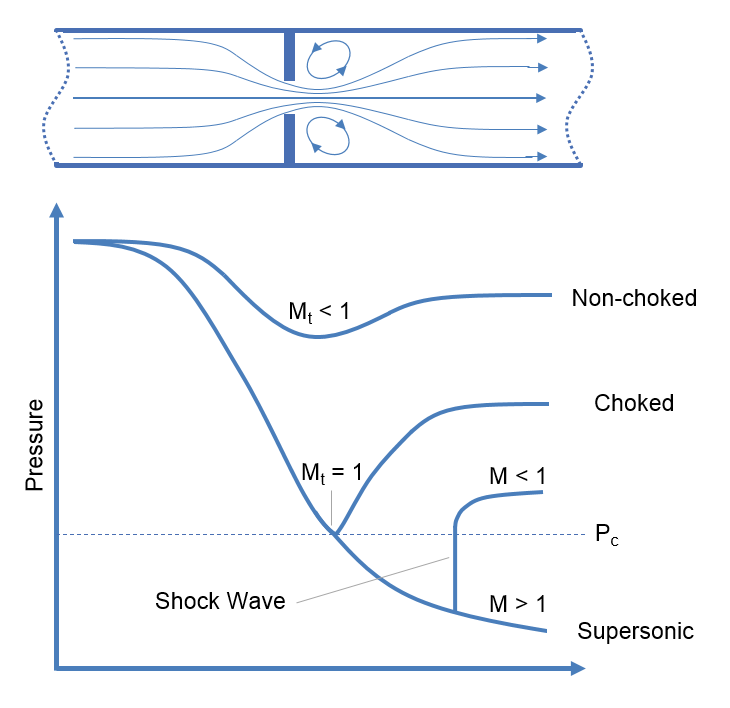Choked flow is a phenomenon that limits the mass flow rate of a compressible fluid flowing through nozzles, orifices and sudden expansions. Generally speaking it is the mass flux after which a further reduction in downstream pressure will not result in an increase in mass flow rate.

Comparing Volumetric Flow Rates for Equivalent Pressure Loss

Standard volumetric flow rates of a fluid are often used to describe the capacity of a vent or pressure relief device. To determine how this capacity compares for another fluid under different pressure and temperature conditions a conversion must be made on the basis of equivalent pressure loss. This article describes the method for calculating the volumetric flow rate of a gas which will give the equivalent pressure drop to another gas through a fixed restriction such as a vent.

Bernoulli's Equation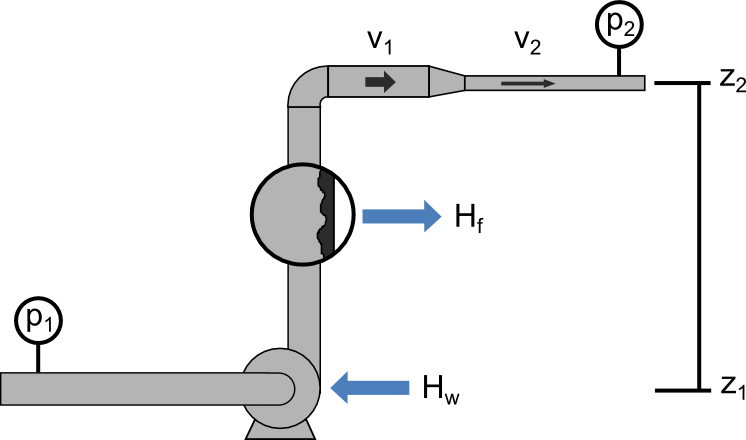Bernoulli’s Principle is an important observation in fluid dynamics which states that for an inviscid flow, an increase in the velocity of the fluid results in a simultaneous decrease in pressure or a decrease in the fluid’s potential energy. This principle is often represented mathematically in the many forms of Bernoulli’s equation. This article presents some useful forms of Bernoulli’s Equations and their simplifying assumptions.

Pressure Loss in Hoses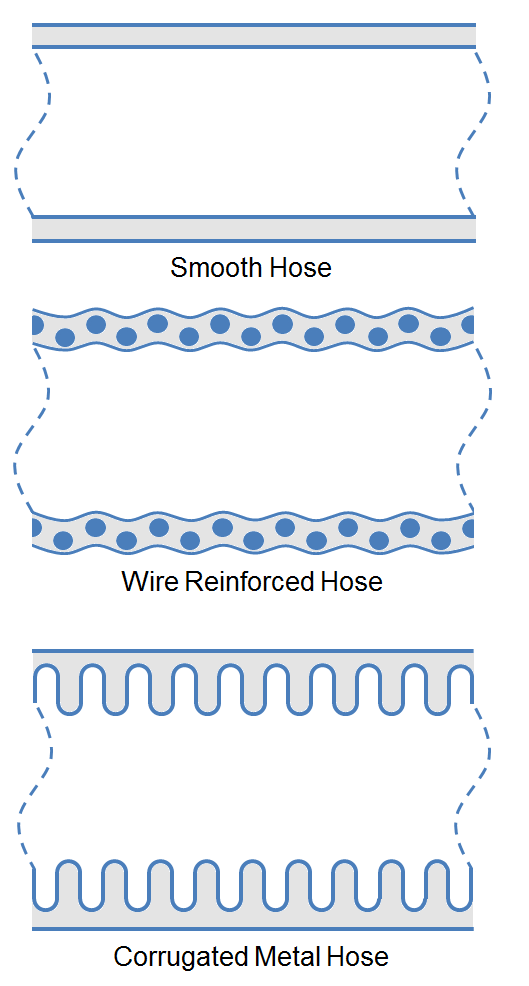The Pressure loss through a hose is often approximated using coarse heuristics, but utilization of more accurate correlations increase the efficiency of pump and piping designs. This article presents more accurate methods to estimate the pressure loss in various type of hoses using multiples of the pipe length. Methods of estimating pressure loss caused by couplings, curves and coiled hose are also detailed.

Pressure Loss from Pipe Entrances and Exits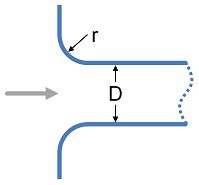When a fluid moves from a tank or vessel into a pipe system or vice versa there are pressure losses. This article provides K-values for pipe entrances and exits of various geometries. These K-values may be used to determine the pressure loss from a fluid flowing through these entrances and exits.

Pressure Loss from Fittings - Expansion and Reduction in Pipe Size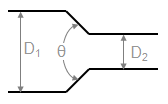This article provides methods to calculate the K-value (Resistance Coefficient) for determining the pressure loss cause by changes in the area of a fluid flow path. These types of pressure drops are highly dependent on the geometry and are not usually covered in simple pressure loss estimation schemes (such as a single k-value, equivalent length etc.)

Pressure Loss from Fittings - 3K Method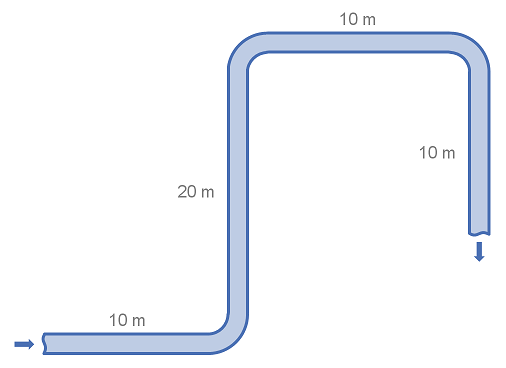Fittings such as elbows, tees, valves and reducers represent a significant component of the pressure loss in most pipe systems. This article details the calculation of pressure losses through pipe fittings and some minor equipment using the 3K method.

Pressure Loss from Fittings - 2K Method

Fittings such as elbows, tees, valves and reducers represent a significant component of the pressure loss in most pipe systems. This article details the calculation of pressure losses through pipe fittings and some minor equipment using the 2K method.

Pressure Loss from Fittings - Excess Head (K) Method

Fittings such as elbows, tees, valves and reducers represent a significant component of the pressure loss in most pipe systems. This article details the calculation of pressure losses through pipe fittings and some minor equipment using the K-value method, also known as the Resistance Coefficient, Velocity Head, Excess Head or Crane method.